## Wednesday, August 15, 2007

### Irodov Problem 1.82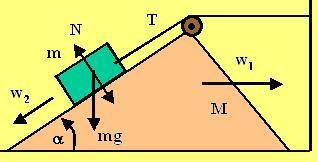The body m pushes the body M backwards. As the body M is pushed backwards, m is forced to slide down the incline on M. Let the tension in the string be T and let the normal reaction between the surfaces be N. Further let the acceleration of body M be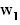and let the acceleration of body m with respect to an observer on body M bealong the direction down the inclined plane.

Relation betweenand: The relation between the two acceleration can be seen in the figure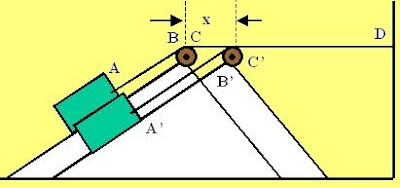below. As seen in the figure, AB and CD are two section of the string before and after the pulley. After the mass M and hence the pulley moves back by x units the length CD shortens to C'D = CD-x. Since, the total length of the string AB + CD is to remain constant AB must extend to A'B' = AB + x. In other words if the mass M moves x units towards the wall, the mass m slides the same x units on the inclined plane. Thus, we have,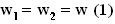Forces on mass m : We will resolve the forces acting on m in the parallel and perpendicular direction to the incline. This mass experiences two kinds of i) accelerations,as it rides along with mass M and ii) its accelerationas it slides on the incline relative to M. The net acceleration is the summation of these two accelerations. From (1) however, the magnitude of both these accelerations is the same.In the direction perpendicular to the incline, there are twp forces acting on the body, i) the component of gravityand ii) the normal reaction N from the surface of mass M. The component of the net acceleration of mass m along the perpendicular direction is given by,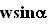as shown in the figure. Thus we have,In the direction parallel to the incline, there are two forces acting on the mass m, i) the tension in the string T and ii) the component of force of gravitypulling it down the incline. The component of net acceleration along this direction is given byas shown in the figure. Thus, we have,Forces on mass M : For this problem we need consider only the forces acting in the horizontal direction - this is shown in the figure.There are three forces acting on the mass M that effect its motion in the horizontal direction, i) the normal reaction from mass m and ii) the tensions of magnitude T in the parts of the string after and before the pulley directed along the direction of the string. The component of tension in the part string connecting the mass m and the pulley is given by. The mass M accelerates at a rate w towards the wall. Thus we have,Now we have all the information needed to solve for w.

From (2) and (4),From (3) and (5) we have,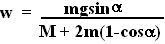Hi,
You have done a gr8 job by posting these solutions.
I was trying to solve this problem,
and i got a different solution. The difference comes due to equation 3 that you are using. You assumed that acceleration along the incline is w2=w. Then why there is 1-cos factor coming there.

Thanks

Krishna Kant Chintalapudi said...

Suppose that you are walking in a bus with a speed v2 relative to the bus and the bus itself is moving with a speed v1 in the opposite direction, your speed as seen by an observer standing on the road will be v1-v2.

In this problem, the mass is accelerating down the incline at a rate w relative to M, but then M itself is moving moving horizontally backwards at a rate w . The net acceleration of the mass as seen by a stationary observer is the sum of these two motions. This is shown in the figure and explained beside it.